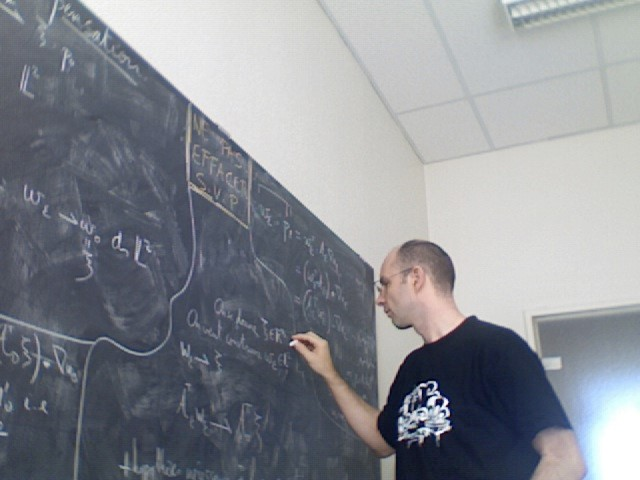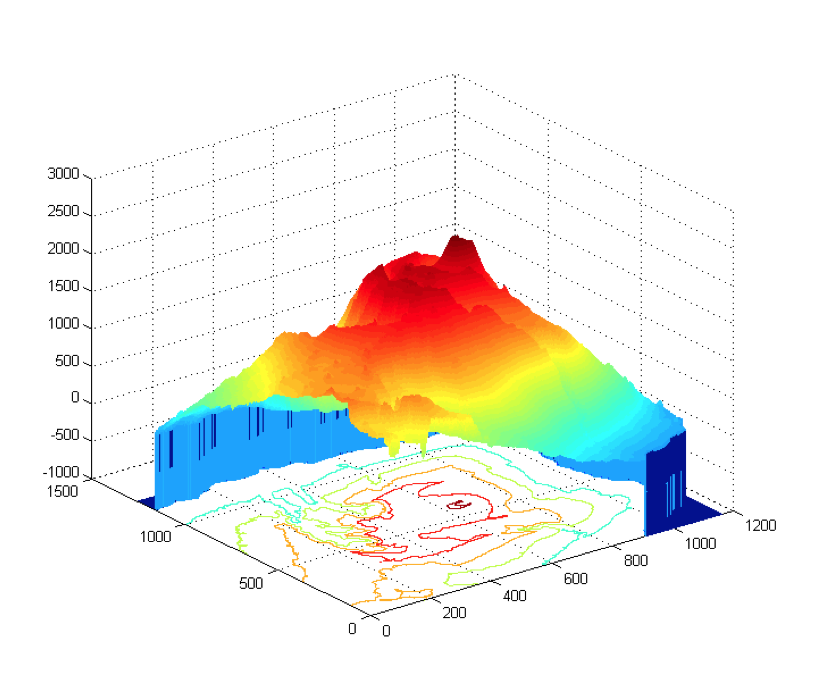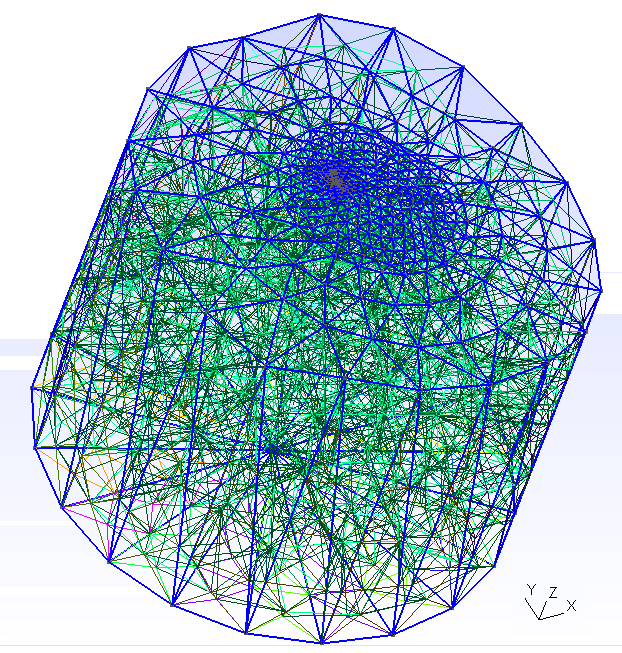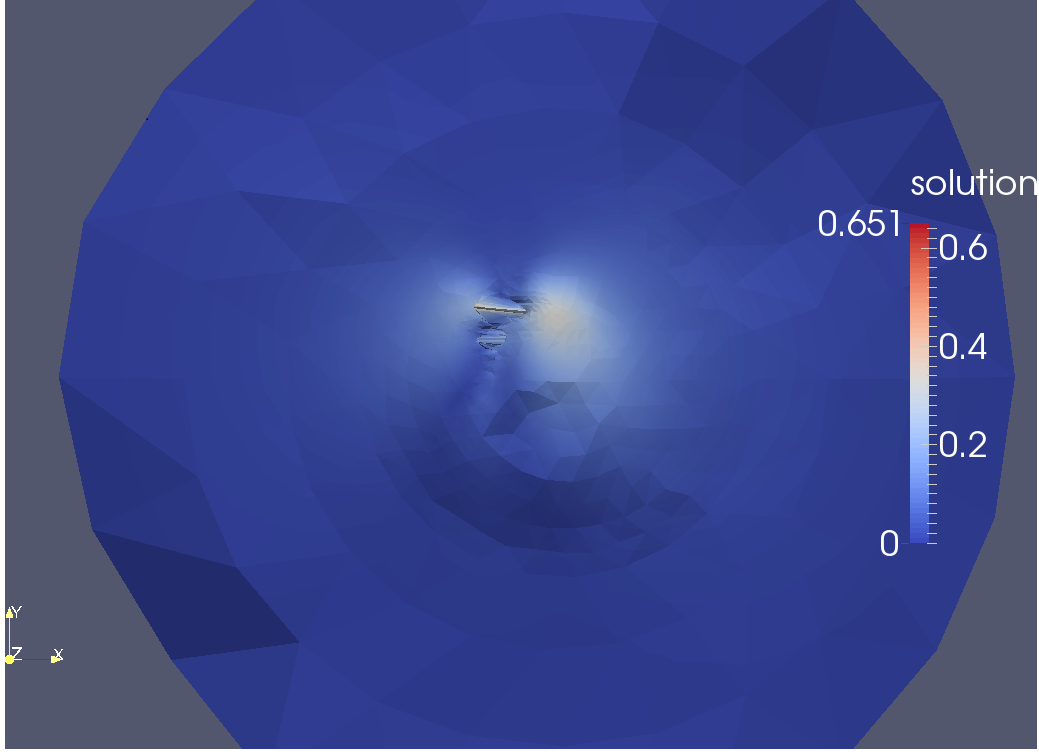# Olivier BodartMaître de Conférences
Habilité à diriger des recherches

Institut Camille Jordan
&
Département de Mathématiques de l'UJM
Courrier électronique (remplacer _AT_ par @)

# Activités de recherche

## EQUATIONS AUX DERIVEES PARTIELLES

### Elasticité - Applications à la volcanologie

• Méthodes de domaines fictifs pour les géométries avec fissures (3D)
• Problèmes inverses (identification de fracture, contrôle optimal)

### Analyse Asymptotique - Domaines à frontière oscillante

• Écoulements stationnaires le long de parois rugueuses
• Comportement limite des solutions
• Construction de lois de paroi
• Méthodes de décomposition de domaines pour approcher numériquement les solutions

### Contrôle des EDP

• Insensibilisation de fonctionnelles
• Contrôle de systèmes paraboliques non-linéaires

## STATISTIQUES - Estimation d'ensembles

• Estimation de supports (multi-dimensionnels) de densité de probabilité
• Estimation d'espérances de Fréchet sur des échantillons supportés sur des variétés# Publications

• O. BODART, V. CAYOL, F. DABAGHI, J. KOKO. An inverse problem in an elastic domain with a crack : a fictitious domain approach. (Submitted - Soumis).
• O. BODART, A. CHORFI, J. KOKO. A fictitious domain decomposition method for a nonlinear bonded structure. Mathematics and Computers in Simulation. (In press - A paraître).
• O. BODART, V. CAYOL, F. DABAGHI, J. KOKO. Fictitious Domain Method for an Inverse Problem in Volcanoes. In R. D. Haynes, S. MacLachlan, X.-C. Cai, L. Halpern, H. H. Kim, A. Klawonn, and O. Widlund, editors, Domain Decomposition Methods in Science and Engineering XXV, volume 138 of Lecture Notes in Computational Science and Engineering, pp. 401-408. Springer Verlag, 2020.
• O. BODART, A. CHORFI, J. KOKO. A Domain Decomposition Method for a Geological Crack. In R. D. Haynes, S. MacLachlan, X.-C. Cai, L. Halpern, H. H. Kim, A. Klawonn, and O. Widlund, editors, Domain Decomposition Methods in Science and Engineering XXV, volume 138 of Lecture Notes in Computational Science and Engineering, pp. 401-408. Springer Verlag, 2020.
• C. AARON, O. BODART. Convergence rates for estimators of geodesic distances and Fréchet expectations. Journal of Applied Probability, 55 (4), pp.1001–1013, 2018.
• Y. AMIRAT, O. BODART, G. CHECHCKIN, A. PIATNITSKI. Asymptotics of a spectral-sieve problem. Journ. of Mathematical Analysis and Applications, 425 (2), pp.1652–1671, 2016.
• C. AARON, O. BODART. Local convex hull support and boundary estimation. Journal of Multivariate Analysis, 147, 82–101, 2016.
• O. BODART, V. CAYOL, S. COURT, J. KOKO. XFEM-based fictitious domain method for linear elasticity model with crack. SIAM Journal on Scientific Computing, 38 (2), pp. B219–B246, 2016.
• V. CAYOL, T. CATRY, L. MICHON, M. CHAPUT, V. FAMIN, O. BODART, J.-L. FROGER, C. ROMAGNOLI. Sheared sheet intrusions as a mechanism for lateral flank displacement on basaltic volcanoes: Applications to Réunion Island volcanoes. Journal of Geophysical Research - Solid Earth, 119(10), pp.7607–7635, 2014.
• Y. AMIRAT, O. BODART, U. DE MAIO, A. GAUDIELLO. Effective boundary condition for Stokes flow over a very rough surface. Journal of Differential Equations, 254(8), pp.3395–3430, 2013.
• Y. AMIRAT, O. BODART, G. CHECHCKIN, A. PIATNITSKI. Boundary homogenization in domains with randomly oscillating boundary. Stochastic Processes and Applications, 121, pp.1–23, 2011.
• Y. AMIRAT, O. BODART. On a boundary value problem in an unbounded domain for stationary Stokes equations with interface condition. Applicable Analysis, X89 (1), pp.29–47 (2010).
• Y. AMIRAT, O. BODART, U. DE MAIO,, A. GAUDIELLO. Asymptotic approximation of the solution of Stokes equations in a domain with highly oscillating boundary. Ann. Univ. Ferrara Sez VII Sci. Mat., 53 (2), pp. 135–148 (2007).
• O. BODART, M. GONZALEZ-BURGOS, R. PEREZ-GARCIA. Insensitizing controls for a heat equation with a nonlinear term involving the state and the gradient. Nonlinear Analysis Theory, Methods and Applications, 57 (5–6) pp. 687–711 (2004).
• O. BODART, M. GONZALEZ-BURGOS, R. PEREZ-GARCIA. Existence Of Insensitizing Controls For A Semilinear Heat Equation With A Superlinear Nonlinearity. Communications in Partial Differential Equations, 29 (7–8) pp. 1017-1050 (2004).
• O. BODART, M. GONZALEZ-BURGOS, R. PEREZ-GARCIA. A local result on Insensitizing controls for a semilinear heat equation with nonlinear boundary Fourier conditions. SIAM Journal on Control and Optimization, 43 (3), pp. 955–969 (2004).
• Y. AMIRAT, O. BODART, U. DE MAIO, A. GAUDIELLO. Asymptotic approximation of the solution of the Laplace equation in a domain with highly oscillating boundary. SIAM Journal for Mathematical Analysis, 25 (6), pp. 1598–1616 (2004).
• Y. AMIRAT, O. BODART. Numerical approximation of laminar flows over rough walls with sharp asperities.Numerical approximation of laminar flows over rough walls with sharp asperities. Journal of Computational and Applied Mathematics, 164–165, pp. 25–38 (2004).
• O. BODART, M. GONZALEZ-BURGOS, R. PEREZ-GARCIA. Insensitizing controls for a semilinear heat equation with a superlinear nonlinearity.Insensitizing controls for a semilinear heat equation with a superlinear nonlinearity. C.R. Acad. Sci., Série I, 335 (8), pp. 677–682 (2002).
• O. BODART, A. V. BOURREAU, R. TOUZANI. Numerical investigation of optimal control of induction heating processes.Numerical investigation of optimal control of induction heating processes. Applied Mathematical Modelling, 25, pp. 697–712 (2001).
• Y. AMIRAT, O. BODART. Boundary layer correctors for the solution of Laplace equation in a domain with oscillating boundary.Boundary layer correctors for the solution of Laplace equation in a domain with oscillating boundary. Journal for Analysis and its Applications, 20 (4), pp. 929-940 (2001).
• O. BODART, P. DEMEESTERE. Boundary control of the finite time blow-up of the solution of a combustion equation. Advances in Mathematical Sciences and Applications, 9 (2), pp. 839–850 (1999).
• O. BODART, P. DEMEESTERE. Sentinels for the identification of un unknown boundary. M3AS, 7 (6), pp. 871–885 (1997).
• O. BODART, P. DEMEESTERE. Contrôle frontière de l'explosion en temps fini de la solution d'une équation de combustion. C.R. Acad. Sci., Série I, 325 (8), pp. 841–845 (1997).
• O. BODART. Existence of approximate controls for a semilinear Laplace equation. Inverse Problems, pp. 27–33 (1996).
• O. BODART, M. ZINSMEISTER. Quelques résultats sur la dimension de Hausdorff de l'ensemble de Julia des polynômes quadratiques. Fundamenta Mathematicae, 151, pp. 121–137 (1996).
• O. BODART, C. FABRE. Controls insensitizing the norm of a semilinear heat equation. Journal of Mathematical Analysis and Applications, 195, pp. 658–683 (1995).
• O. BODART, J. KERNEVEZ, T. MäNNIKKö. Numerical methods to compute sentinels for distributed systems. In J. YVON J. HENRY (eds.), Proccedings of the 16th IFIP TC7 conference on System modelling and Optimization. Springer Verlag, Paris (1994).
• O. BODART, T. MäNNIKKö. Parameter identification with the method of sentinels. In NEITTAANMäKI (ed.), Workshop on Optimization and Control, pp. 97–107. Ber. Univ. Jyväskylä Inst., Jyväskylä (Finland) (1993).
• O. BODART, C. FABRE. Contrôle insensibilisant la norme de la solution d'une équation de la chaleur semi-linéaire. C.R. Acad. Sci., Série I, 316(8), pp. 789–794 (1993).
• O. BODART, J. KERNEVEZ T. MäNNIKKö. Sentinels for distributed environmental systems. In Eleventh IASTED International Conference on Modelling, Identification and Control, pp. 219–222. Innsbruck (Austria) (1992).
• O. BODART J.-P. KERNEVEZ. Sentinels in rivers. In Jornadas Hispano-Francesas sobre Control de Sistemos Distribuidos, pp. 69–76. Málaga (Sp.) (1990).